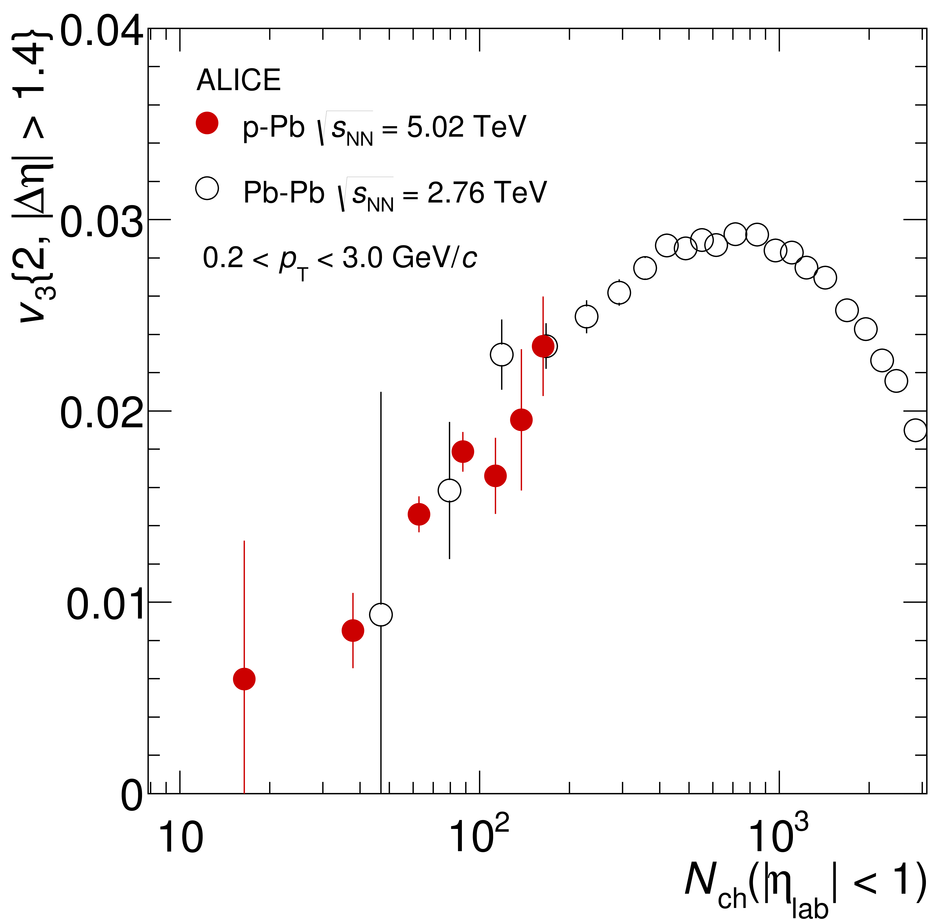Multi-particle azimuthal correlations in p-Pb and Pb-Pb collisions at the CERN Large Hadron Collider

Measurements of multi-particle azimuthal correlations (cumulants) for charged particles in p-Pb and Pb-Pb collisions are presented. They help address the question of whether there is evidence for global, flow-like, azimuthal correlations in the p-Pb system. Comparisons are made to measurements from the larger Pb-Pb system, where such evidence is established. In particular, the second harmonic two-particle cumulants are found to decrease with multiplicity, characteristic of a dominance of few-particle correlations in p-Pb collisions. However, when a $|\Delta \eta|$ gap is placed to suppress such correlations, the two-particle cumulants begin to rise at high-multiplicity, indicating the presence of global azimuthal correlations. The Pb-Pb values are higher than the p-Pb values at similar multiplicities. In both systems, the second harmonic four-particle cumulants exhibit a transition from positive to negative values when the multiplicity increases. The negative values allow for a measurement of $v_{2}\{4\}$ to be made, which is found to be higher in Pb-Pb collisions at similar multiplicities. The second harmonic six-particle cumulants are also found to be higher in Pb-Pb collisions. In Pb-Pb collisions, we generally find $v_{2}\{4\} \simeq v_{2}\{6\}\neq 0$ which is indicative of a Bessel-Gaussian function for the $v_{2}$ distribution. For very high-multiplicity Pb-Pb collisions, we observe that the four- and six-particle cumulants become consistent with 0. Finally, third harmonic two-particle cumulants in p-Pb and Pb-Pb are measured. These are found to be similar for overlapping multiplicities, when a $|\Delta\eta| > 1.4$ gap is placed.

Figures

Figure 1

 Mid-rapidity ($|\eta| < 1$) measurements of $c_{2}\{2\}$ as a function of multiplicity for p-Pb collisions. Only statistical errors are shown as these dominate the uncertainty.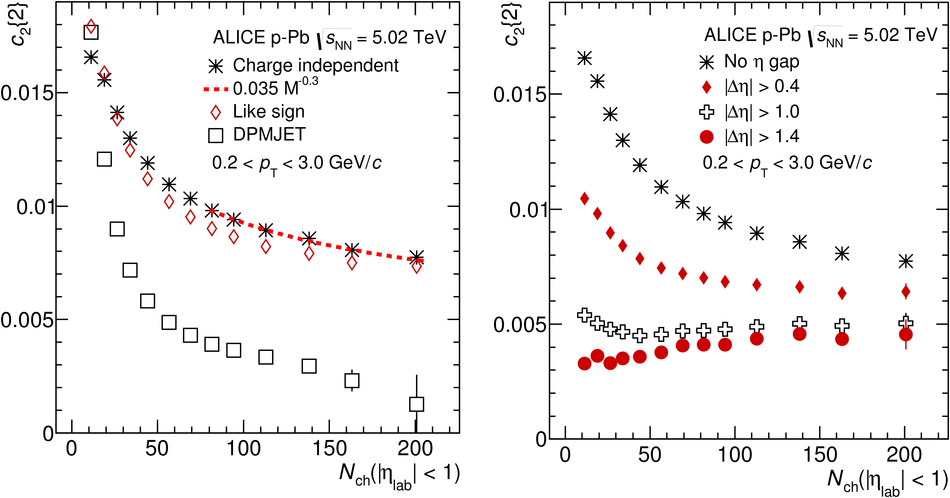Figure 2

 Mid-rapidity ($|\eta| < 1$) measurements of $c_{2}\{2\}$ as a function of multiplicity in Pb-Pb collisions. Only statistical errors are shown as these dominate the uncertainty.Figure 3

 Comparison of $c_{2}\{2\}$ with $|\Delta \eta| > 1.4$ for p-Pb and Pb-Pb collisions. Only statistical errors are shown as these dominate the uncertainty.Figure 4

 Mid-rapidity ($|\eta| < 1$) measurements of $c_{2}\{4\}$ as a function of multiplicity for p-Pb collisions. Only statistical errors are shown as these dominate the uncertainty. The right panel shows a zoomed in version of the solid points in the left panel.Figure 5

 Top panel: Mid-rapidity ($|\eta| < 1$) measurements of $c_{2}\{4\}$ as a function of multiplicity for Pb-Pb collisions. Bottom panel: Comparison of $c_{2}\{4\}$ for $p$-Pb and Pb-Pb collisions. Only statistical errors are shown as these dominate the uncertainty.Figure 6

 Comparison of mid-rapidity ($|\eta| < 1$) $c_{2}\{6\}$ for p-Pb and Pb-Pb collisions. Only statistical errors are shown as these dominate the uncertainty.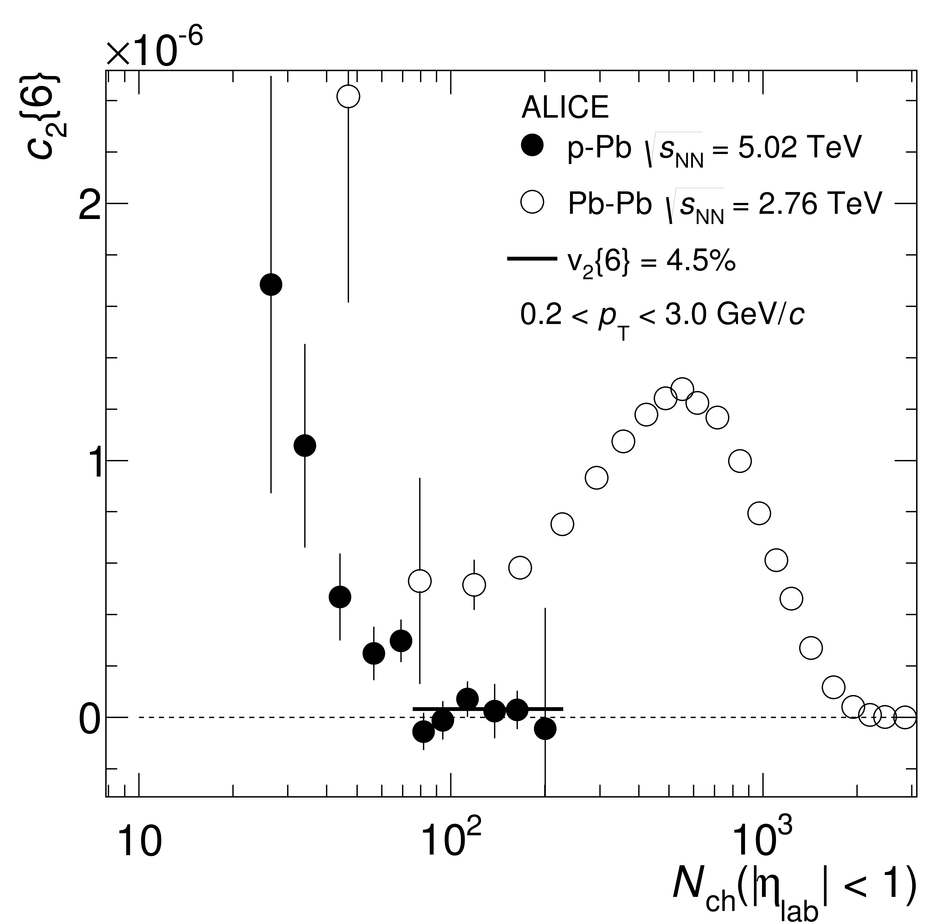Figure 7

 Comparison of $c_{2}\{m\}$ in very high-multiplicity Pb-Pb collisions. Only statistical errors are shown as these dominate the uncertainty.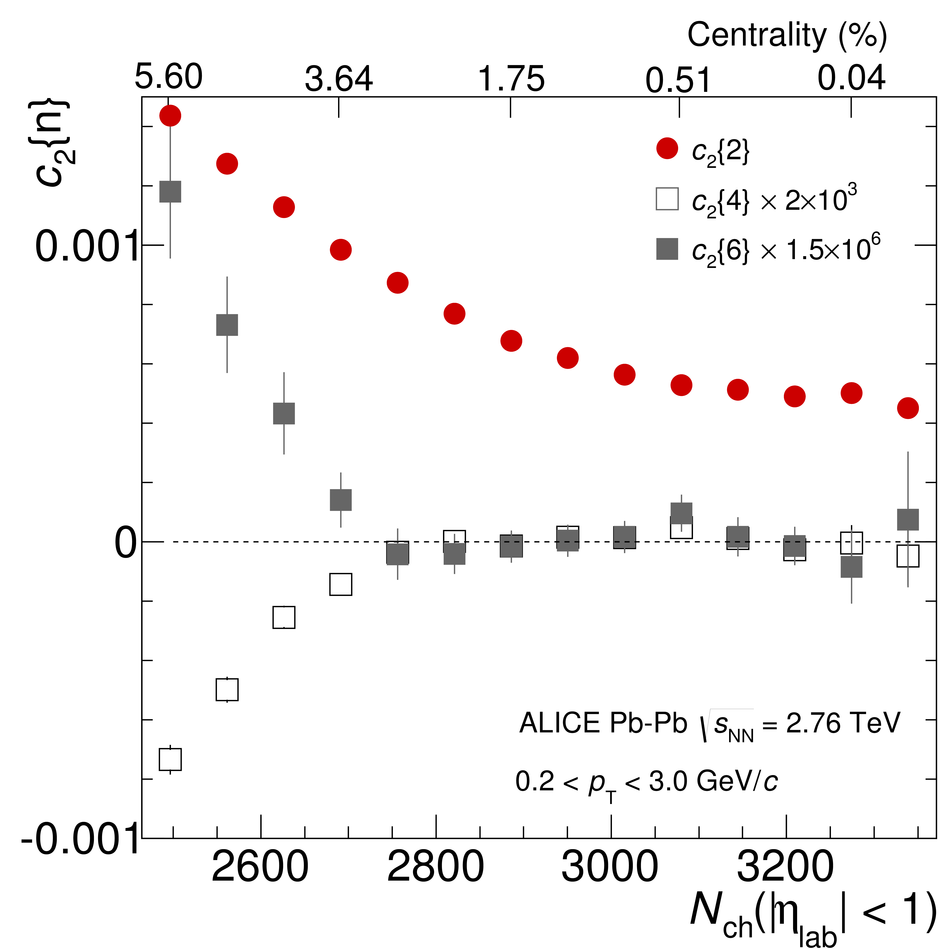Figure 8

 Measurements of $v_{2}\{2\}$, $v_{2}\{4\}$, and $v_{2}\{6\}$ in p-Pb (left panel) and Pb-Pb (right panel) collisions. The measurements of $v_{2}\{2\}$ are obtained with a $|\Delta \eta| > 1.4$ gap. Only statistical errors are shown as these dominate the uncertainty.Figure 9

 Top panel: Measurements of $[ (v_{2}\{2\}^{2}-v_{2}\{4\}^{2})/(v_{2}\{2\}^{2}+v_{2}\{4\}^{2})]^{1/2}$ in p-Pb and Pb-Pb collisions. The measurements of $v_{2}\{2\}$ are obtained with a $|\Delta \eta| > 1.4$ gap. Only statistical errors are shown as these dominate the uncertainty. Bottom panel: $\sigma_{v2} / \langle v_{2} \rangle$ obtained from the same $v_{2}\{2\}$ and $v_{2}\{4\}$ measurements assuming a Bessel-Gaussian distribution.Figure 10

 Third harmonic two-particle cumulants in p-Pb and Pb-Pb collisions. Only statistical errors are shown as these dominate the uncertainty.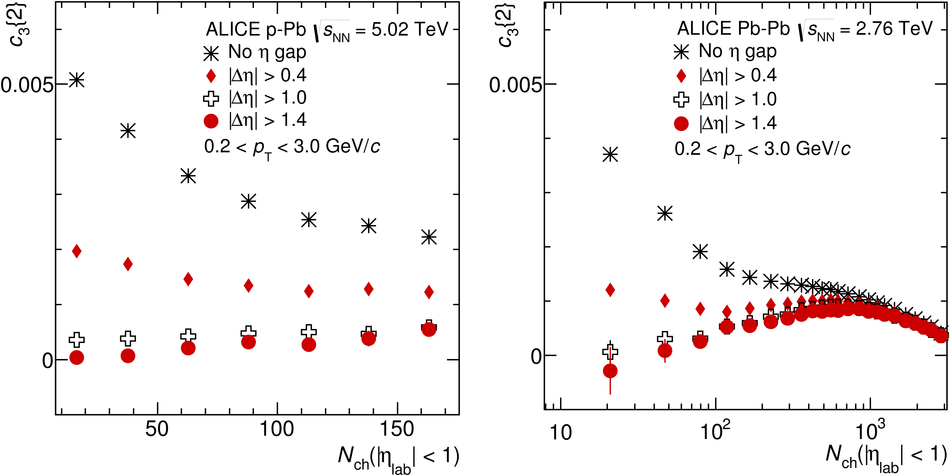Figure 11

 Third harmonic flow coefficients in p-Pb and Pb-Pb collisions. The measurements of $v_{3}\{2\}$ are obtained with a $|\Delta \eta| > 1.4$ gap. Only statistical errors are shown as these dominate the uncertainty.